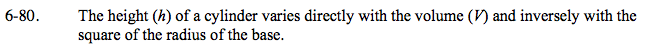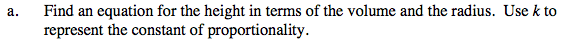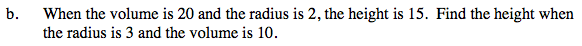### Home > PC > Chapter 6 > Lesson 6.2.1 > Problem6-80

6-80.
1. The height (h) of a cylinder varies directly with the volume (V) and inversely with the square of the radius of the base. Homework Help ✎

1. Find an equation for the height in terms of the volume and the radius. Use k to represent the constant of proportionality.

2. When the volume is 20 and the radius is 2, the height is 15. Find the height when the radius is 3 and the volume is 10.$h=\frac{kV}{r^{2}}$